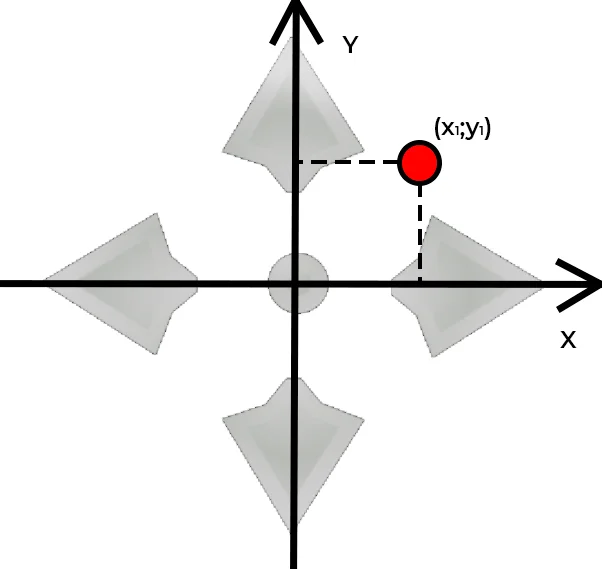# Sensory variables for the TRIK controller

Sensor variables are reserved variables for getting readings of the sensors connected to the corresponding ports.
These values can be assigned to variables and used in mathematical expressions.
Variable changes can be tracked as you go through the program in the variable table.

## accelerometer

An array of three elements containing accelerometer readings on the X, Y, and Z axis, respectively.

#### Example

x = accelerometer; -- X-axis accelerometer readings
y = accelerometer; -- Y-axis accelerometer readings
z = accelerometer; -- Z-axis accelerometer readings

## colorSensorR, colorSensorG, colorSensorB

The color sensor readings by the camcorder in the center of the frame are the intensity of red, green, and blue, respectively. They are only updated when the color sensor is turned on and initialized.

From 0 to 255.

#### Example

r = colorSensorR; -- red intensity
g = colorSensorG; -- green intensity
b = colorSensorB; -- blue intensityThe coordinates of pressing the active areas on the remote control.
Each variable stores an array of two elements corresponding to the coordinate of the click point.#### Possible values

From (-100, -100) to (100, 100), (-100, -100) - top left corner of the active area. (-101, -101) - if there is no click.

## gyroscope

An array of three elements containing the gyroscope readings on the X, Y, and Z-axis respectively.

#### Пример

x = gyroscope; -- X-axis gyroscope readings
y = gyroscope; -- Y-axis gyroscope readings
z = gyroscope; -- Z-axis gyroscope readings

## lineSensor

An array of three elements containing camera readings inline sensor mode. The values are updated when the sensor is on and initialized.
 ​ ​ lineSensor The deviation of the line from the screen center from -100 (leftmost line position in the frame) to 100 (rightmost line position). lineSensor Crossing probability is defined as the percentage of points inside the horizontal stripe in the center of the frame that is recognized as a line, from 0 (no such points) to 100 (all points in the horizontal strip are recognized as a line). lineSensor Relative line size, that is, the number of points in the frame defined as a line, from 0 (no line in the frame) to 100 (the whole frame is recognized as a line).